# Unitary matrices

(diff) ← Older revision | Latest revision (diff) | Newer revision → (diff)
A unitary matrix is a complex matrix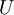$U$ satisfying the condition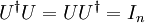$U^\dagger U = UU^\dagger = I_n\,$
where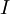$I$ is the identity matrix and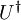$U^\dagger$ is the conjugate transpose (also called the Hermitian adjoint) of$U$. Note this condition says that a matrix$U$ is unitary if and only if it has an inverse which is equal to its conjugate transpose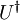$U^\dagger \,$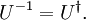$U^{-1} = U^\dagger.$